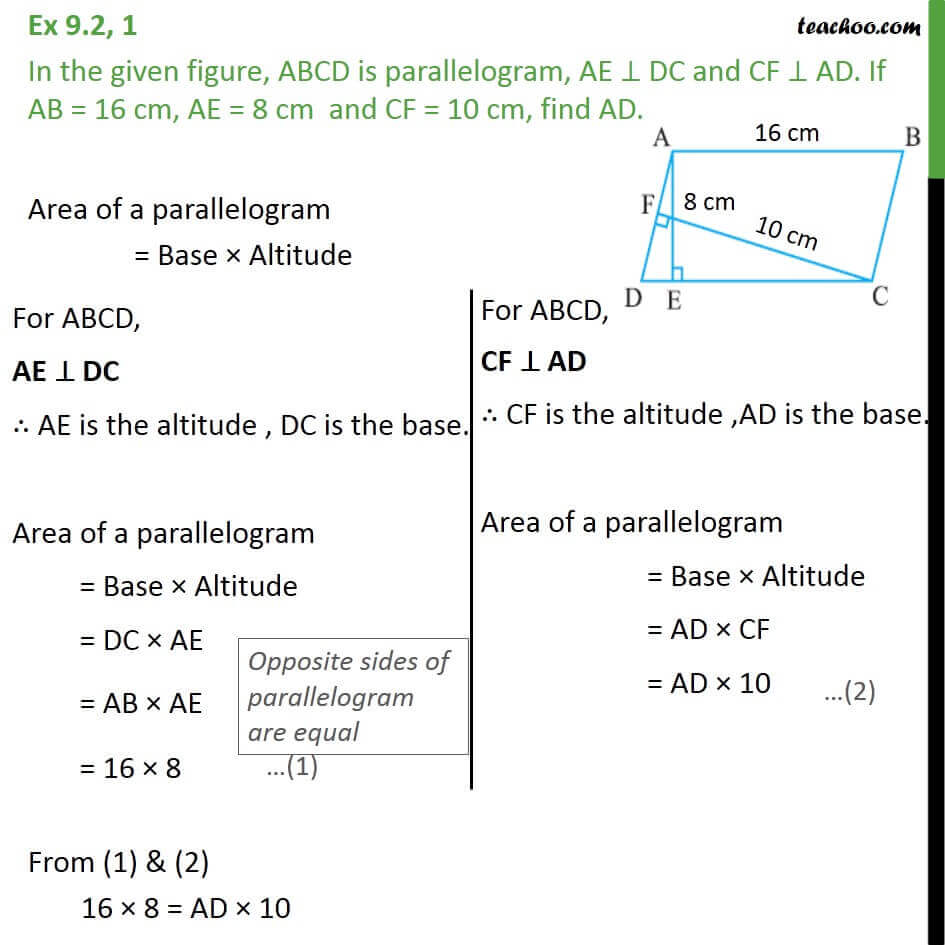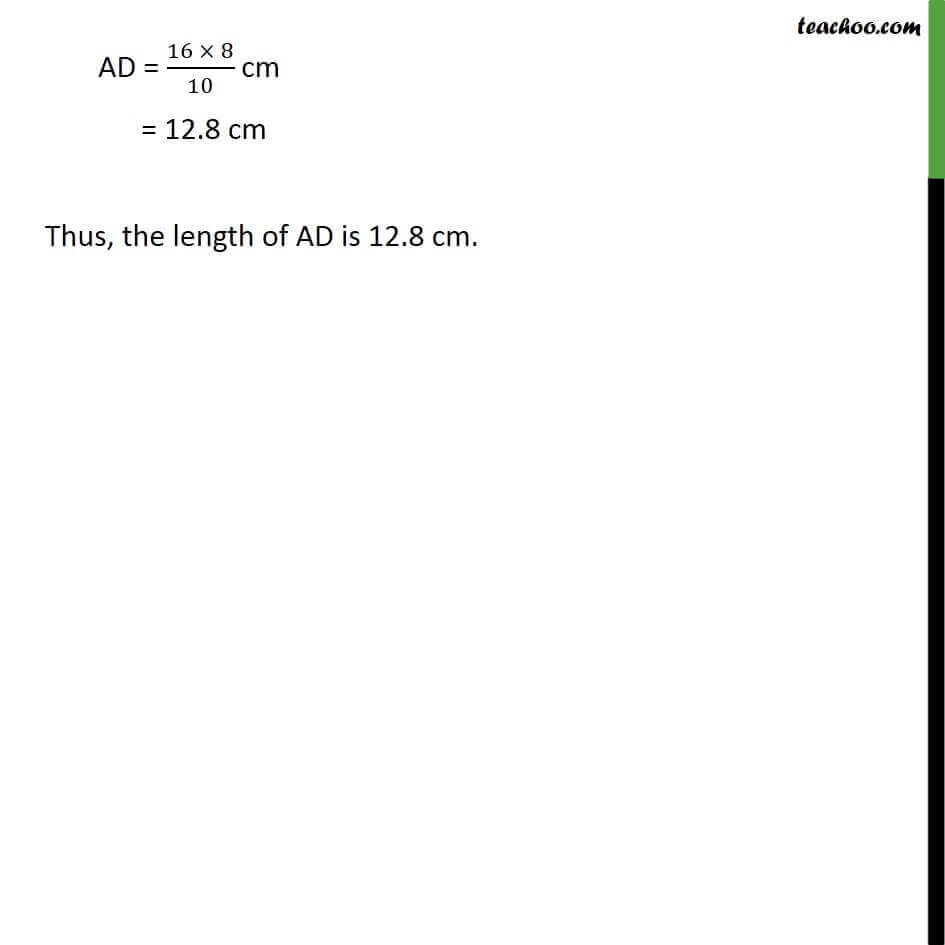Subscribe to our Youtube Channel - https://you.tube/teachoo

1. Chapter 9 Class 9 Areas of Parallelograms and Triangles
2. Serial order wise
3. Ex 9.2

Transcript

Ex 9.2, 1 In the given figure, ABCD is parallelogram, AE DC and CF AD. If AB = 16 cm, AE = 8 cm and CF = 10 cm, find AD. Area of a parallelogram = Base Altitude From (1) & (2) 16 8 = AD 10 AD = (16 8)/10 cm = 12.8 cm Thus, the length of AD is 12.8 cm.

Ex 9.2